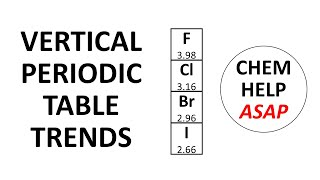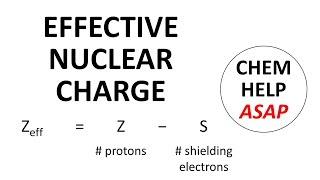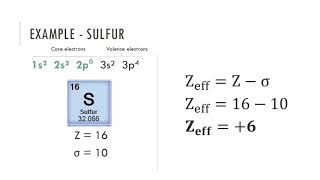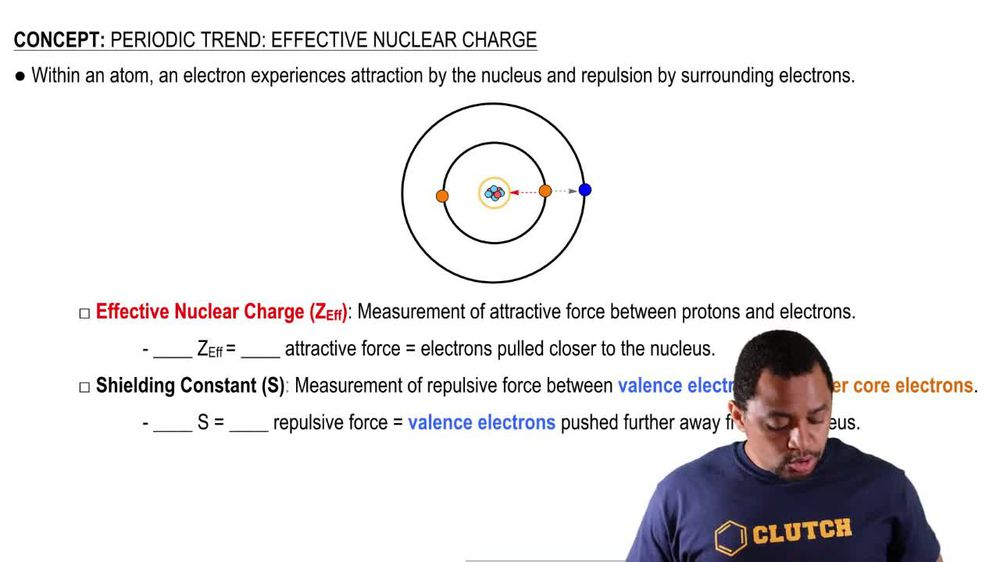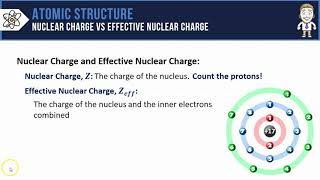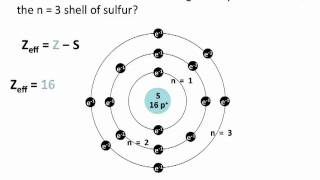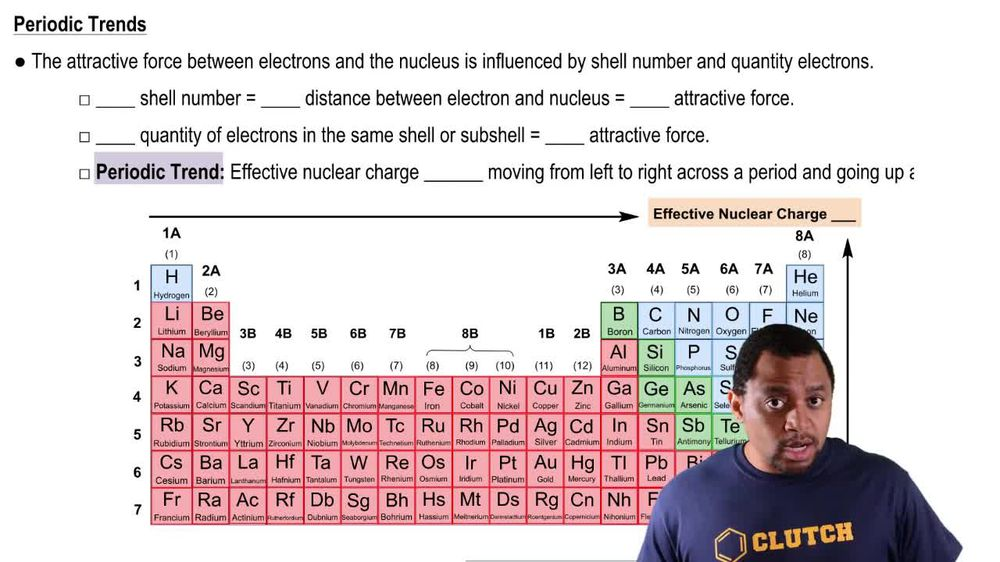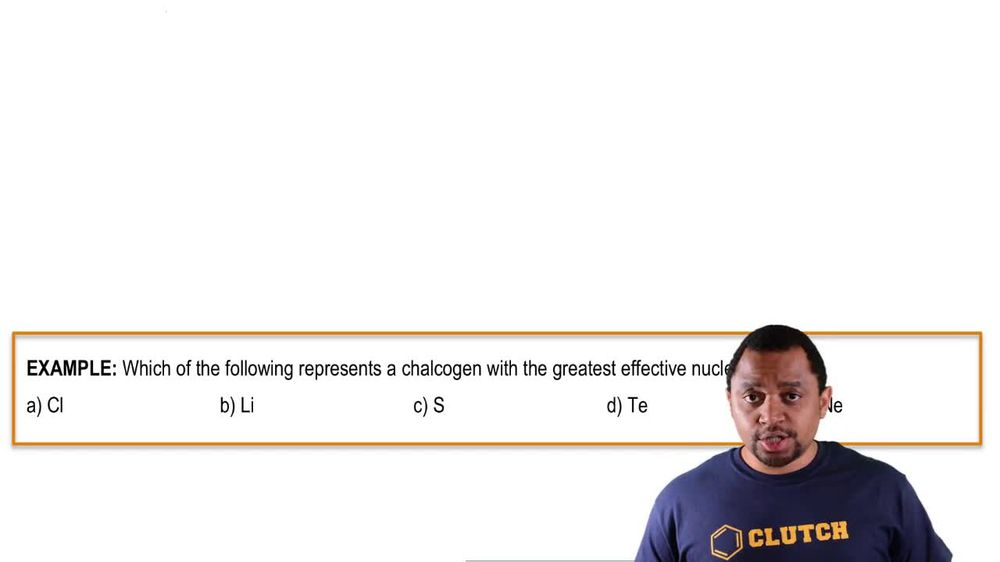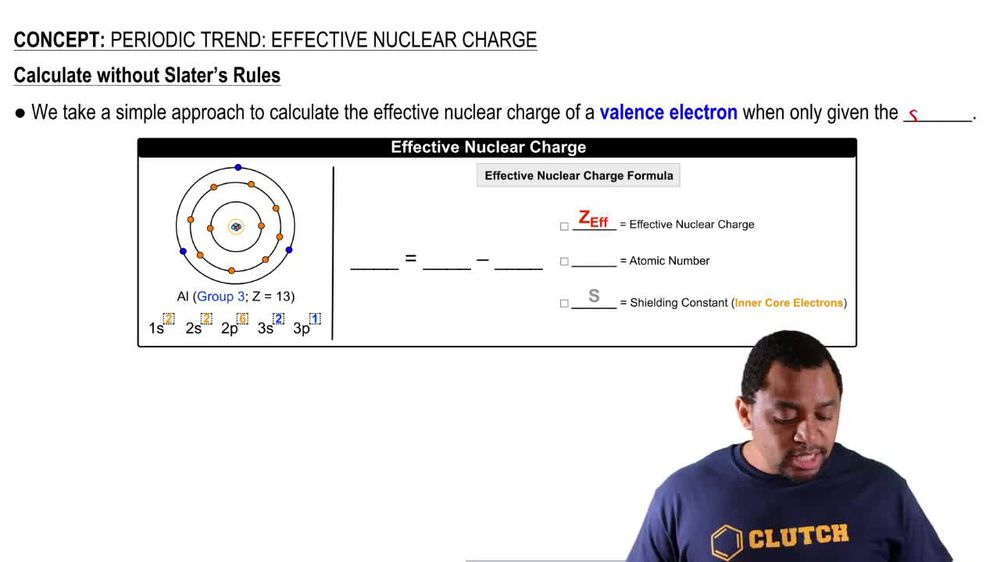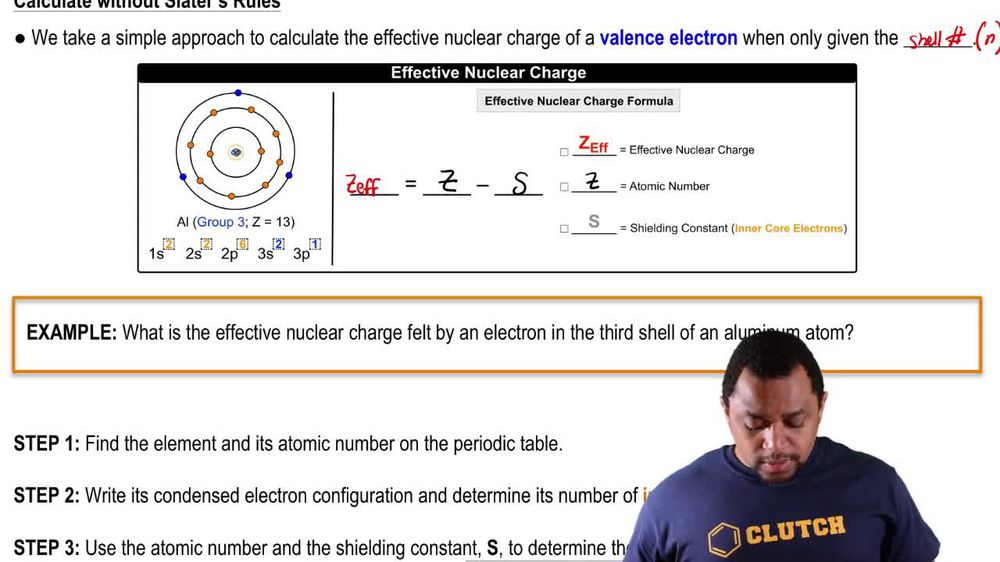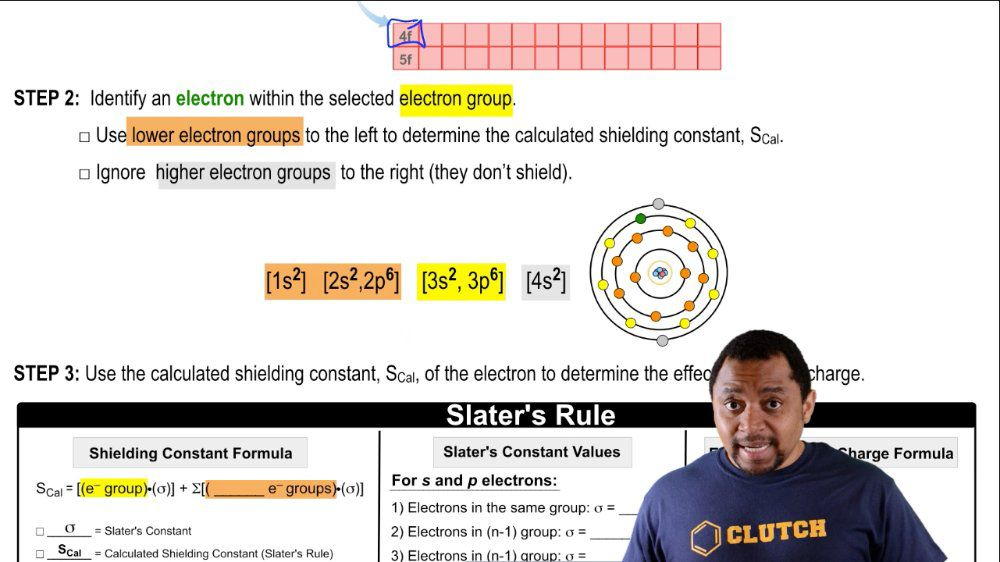Start typing, then use the up and down arrows to select an option from the list.
1. 10. Periodic Properties of the Elements2. Periodic Trend: Effective Nuclear Charge# Periodic Trend: Effective Nuclear Charge

by Jules Bruno
246 views
1
0
here we take a simple approach to calculate the effective nuclear charge of a valence electron. When Onley given the shell number remember your shell number uses the variable end which stands for the principle quantum number. Now here we have the atomic view off the aluminum atom Aluminum is in group three A. It has an atomic number 13. Here we say that it is one s two to s 22 p 63 s, 23 p one For its electron configuration, it's effective nuclear charge formula, which is simply be effective. Nuclear charge, which is e f. Equals the atomic number of the element minus. It's shielding Constant s Now hear the shooting constant could be seen as the inner core electrons for the given element. So we use this simplified version of the effective nuclear charge when we only have the shell number